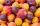Diophantus

We know little about this Greek mathematician from Alexandria, except that he lived around 3rd century A.D. Thanks to an admirer of his, who described his life by means of an algebraic riddle, we know at least something about his life.
Diophantus's youth lasted 1/6 of his life. He had his first beard in the next 1/12 of his life. At the end of the following 1/7 of his life Diophantus got married. Five years from then his son was born. His son lived exactly 1/2 of Diophantus's life. Diophantus died 4 years after the death of his son.

How long did Diophantus live? [Diophantus epitaf]

Result

x =  84

Solution:1/6* x + 1/12 * x + 1/7 * x + 5 + 1/2 * x + 4 = x

9x = 756

x = 84

Calculated by our simple equation calculator.

Leave us a comment of this math problem and its solution (i.e. if it is still somewhat unclear...):Be the first to comment!To solve this verbal math problem are needed these knowledge from mathematics:

Need help calculate sum, simplify or multiply fractions? Try our fraction calculator. Do you have a linear equation or system of equations and looking for its solution? Or do you have quadratic equation?

Next similar math problems:

1. Unknown number 6Determine the unknown number, which is by 1.5 greater than its fourth.
2. Unknown number 11That number increased by three equals three times itself?
3. Watching TVOne evening 2/3 students watch TV. Of those students, 3/8 watched a reality show. Of the students that watched the show, 1/4 of them recorded it. What fraction of the students watched and recorded reality tv.
4. HomeworkIn the crate are 18 plums, 27 apricot and 36 nuts. How many pieces of fruit left in the crate when Peter took 8 ninth: 1. nuts 2. apricots 3. fruit 4. drupe
5. A numberA number increased by 7.9 is 8.3
6. Simple equation 9Solve the following equation: -8y+5=-9y+9
7. Find xSolve: if 2(x-1)=14, then x= (solve an equation with one unknown)
8. Equation 15Solve equation with variables on both sides:
9. Evaluate expressionEvaluate expression using BODMAS rule: 1 1/4+1 1/5÷3/5-5/8
10. SickSick Marcel already taken six tablets, which was a quarter of the total number of pills in the pack. How many pills were in the pack?
11. EquationSolve equation and check the result: 1.4x - 3/2 + x - 9,8 = x + 0,4/3 - 7 + 1,6/6
12. Sum of fractionsWhat is the sum of 2/3+3/5?
13. Forest nurseryIn the forest nursery after winter, they found that 1/10 stems died out of them. For them, they land 193 new spruces. How many spruces are in the forest nursery?
14. TeacherTeacher Rem bought 360 pieces of cupcakes for the outreach program of their school. 5/9 of the cupcakes were chocolate flavor and 1/4 wete pandan flavor and the rest were a vanilla flavor. How much more pandan flavor cupcakes than vanilla flavor?
15. Pizza 5You have 2/4 of a pizza and you want to share it equally between 2 people how much pizza does each person get?
16. Equation 11Solve equation: ?
17. SymbolsIf 2*3 = 60 ; 3*4 = 120 and 4*5 = 200, what is 2*5?